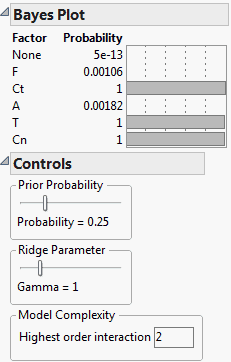Fitting Linear Models > Standard Least Squares Examples > Bayes Plot for Active Factors Example
Publication date: 08/13/2020

# Bayes Plot for Active Factors Example

Suppose that you conduct an experimental design and want to determine which factors are active. You can address this in several ways using JMP. This example illustrates a script that can be used to identify active factors using a Bayesian approach.

1. Select Help > Sample Data Library and open Reactor.jmp.

2. In the Samples > Scripts folder, open the BayesPlotforFactors.jsl sample script.

3. Select Edit > Run Script.

4. Select Y and click Y, Response.

5. Select F, Ct, A, T, and Cn and click X, Factor.

6. Click OK.

Figure 4.24 Bayes Plot for Factor ActivityThe Model Complexity indicates that the highest order interaction to consider is two. Therefore, all possible models that include up to second-order interactions are constructed. Based on the value assigned to Prior Probability, a posterior probability is computed for each of the possible models. The probability for a factor is the sum of the probabilities for each of the models where it was involved.

This approach identifies Ct, T, and Cn as active factors, and A and F as inactive.

If the ridge parameter were zero (not allowed), all the models would be fit by least squares. As the ridge parameter increases, the parameter estimates for any model shrink toward zero. For more information on the ridge parameter, and why it cannot be zero, see Box and Meyer (1993).